Open in App
Not now

# How to resize a tensor in PyTorch?

• Last Updated : 23 Mar, 2022

In this article, we will discuss how to resize a Tensor in Pytorch. Resize allows us to change the size of the tensor. we have multiple methods to resize a tensor in PyTorch. let’s discuss the available methods.

## Method 1: Using view() method

We can resize the tensors in PyTorch by using the view() method. view() method allows us to change the dimension of the tensor but always make sure the total number of elements in a tensor must match before and after resizing tensors. The below syntax is used to resize a tensor.

Syntax: torch.view(shape):

Parameters: updated shape of tensor.

Return: view() method returns a new tensor with the same data as the self tensor but of a different shape.

Example 1:

The following program is to resize 1D tensor in PyTorch using view()

## Python

 `# Import the torch library` `import` `torch`   `# define a tensor` `tens ``=` `torch.Tensor([``10``, ``20``, ``30``, ``40``, ``50``, ``60``])` `print``(``"Original Tensor: "``, tens)`   `# below is the different ways to resize ` `# tensor to 2x3 using view()`   `# Resize tensor to 2x3` `tens_1 ``=` `tens.view(``2``, ``3``)` `print``(``" Tensor After Resize: \n"``, tens_1)`   `# other way resize tensor to 2x3` `tens_2 ``=` `tens.view(``2``, ``-``1``)` `print``(``" Tensor after resize: \n"``, tens_2)`   `# Other way to resize tensor to 2x3` `tens_3 ``=` `tens.view(``-``1``, ``3``)` `print``(``" Tensor after resize: \n"``, tens_3)`

Output: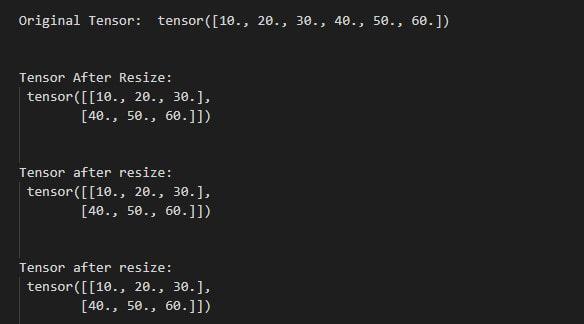Example 2:

The following program is to resize the 2D tensor in PyTorch using view().

## Python

 `# Import the torch library` `import` `torch`   `# define a tensor` `tens ``=` `torch.Tensor([[``1``, ``2``, ``3``], [``4``, ``5``, ``6``], ` `                     ``[``7``, ``8``, ``9``], [``10``, ``11``, ``12``]])` `print``(``"Original Tensor: \n"``, tens)`   `# below is the different ways to use view()`   `# Resize tensor to 3x4` `tens_1 ``=` `tens.view(``2``, ``-``1``)` `print``(``"\n Tensor After Resize: \n"``, tens_1)`   `# other way resize tensor to 3x4` `tens_2 ``=` `tens.view(``-``1``, ``4``)` `print``(``"\n Tensor after resize: \n"``, tens_2)`   `# Other way to resize tensor to 3x4` `tens_3 ``=` `tens.view(``3``, ``-``1``)` `print``(``"\n Tensor after resize: \n"``, tens_3)`

Output: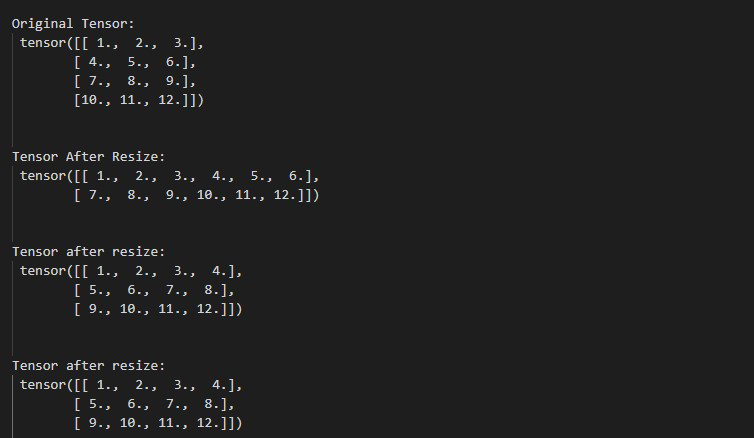## Method 2 : Using reshape() Method

This method is also used to resize the tensors. This method returns a new tensor with a modified size. the below syntax is used to resize the tensor using reshape() method.

Syntax: tensor.reshape( [row,column] )

• row represents the number of rows in the reshaped tensor.
• column represents the number of columns  in the reshaped tensor.

Return: return a resized tensor.

Example 3:

The following program is to know how to resize a 1D tensor to a 2D tensor.

## Python

 `# import torch module` `import` `torch`   `# Define an 1D tensor` `tens ``=` `torch.tensor([``10``, ``20``, ``30``, ``40``, ``50``, ``60``, ``70``, ``80``])`   `# display tensor` `print``(``"\n Original 1D Tensor: "``, tens)`   `# resize this tensor into 2x4` `tens_1 ``=` `tens.reshape([``2``, ``4``])` `print``(``"\n After Resize this Tensor to 2x4 : \n"``, tens_1)`   `# resize this tensor into 4x2` `tens_2 ``=` `tens.reshape([``4``, ``2``])` `print``(``"\n After Resize this Tensor to 4x2 : \n"``, tens_2)`

Output: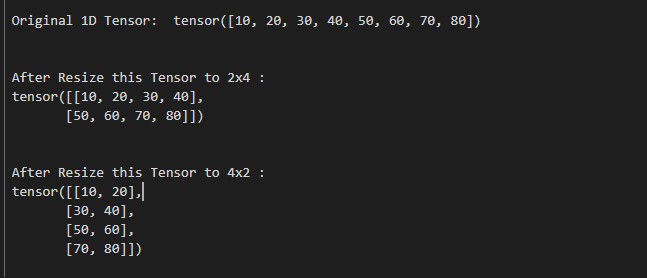Example 4:

The following program is to know how to resize a 2D tensor using reshape() method.

## Python

 `# import torch module` `import` `torch`   `# Define an 2D tensor` `tens ``=` `torch.Tensor([[``1``, ``2``, ``3``], [``4``, ``5``, ``6``],` `                     ``[``7``, ``8``, ``9``], [``10``, ``11``, ``12``]])`   `# display tensor` `print``(``" Original 2D Tensor: \n"``, tens)`   `# resize this tensor to 2x6` `tens_1 ``=` `tens.reshape([``2``, ``6``])` `print``(``"\n After Resize this Tensor to 2x6 : \n"``, tens_1)`   `# resize this tensor into 6x2` `tens_2 ``=` `tens.reshape([``6``, ``2``])` `print``(``"\n After Resize this Tensor to 6x2 : \n"``, tens_2)`

Output: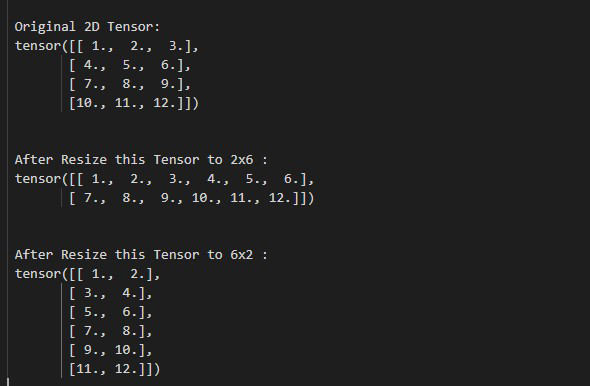## Method 3: Using resize() method

This method is also used to resize tensors in PyTorch and the below syntax helps us to resize the tensor.

Syntax: tensor.resize_(no_of_tensors, no_of_rows, no_of_columns)

Parameters:

• no_of_tensors: represents the total number of tensors to be generated
• no_of_rows: represents the total number of rows in the new resized tensor
• no_of_columns: represents the total number of columns in the new resized tensor

Example 5:

The following program is to understand how to resize the tensor using resize() method.

## Python

 `# import torch module` `import` `torch`   `# Define an 1D tensor` `tens ``=` `torch.Tensor([``11``, ``12``, ``13``, ``14``, ``15``, ``16``, ``17``, ``18``])`   `# display tensor` `print``(``"\n Original 2D Tensor: \n"``, tens)`   `# resize the tensor to 4 tensors.` `# each tensor with 4 rows and 5 columns` `tens_1 ``=` `tens.resize_(``4``, ``4``, ``5``)` `print``(``"\n After resize tensor: \n"``, tens_1)`

Output: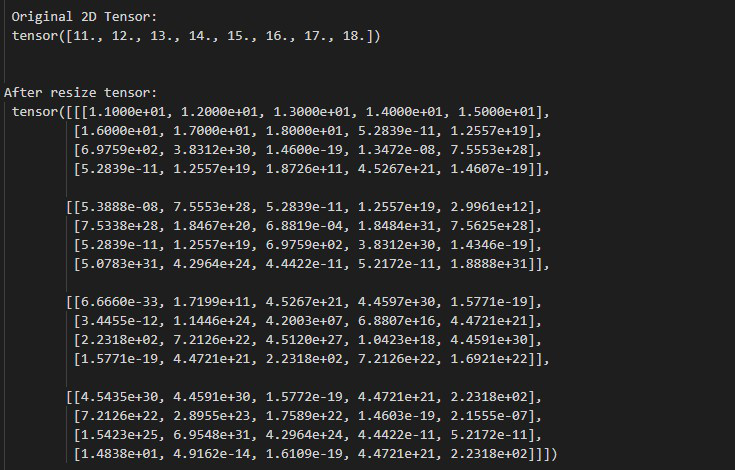## Method 4: Using unsqueeze() method

This is used to resize a tensor by adding new dimensions at given positions. below syntax is used to resize tensor using unsqueeze() method.

Syntax: tensor.unsqueeze(position)

Parameter: position is the dimension index which will start from 0.

Return: It returns a new tensor dimension of size 1 inserted at specific position.

Example 6:

The following program is to resize tensor using unsqueeze() method.

## Python

 `# import torch library` `import` `torch`   `# define a tensor` `tens ``=` `torch.Tensor([``10``, ``20``, ``30``, ``40``, ``50``])`   `# display tensor and it's size` `print``(``"\n Original Tensor: "``, tens)`   `# Squeeze the tensor in dimension 1` `tens_1 ``=` `torch.unsqueeze(tens, dim``=``1``)` `print``(``"\n After resize tensor to 5x1: \n"``, tens_1)`

Output: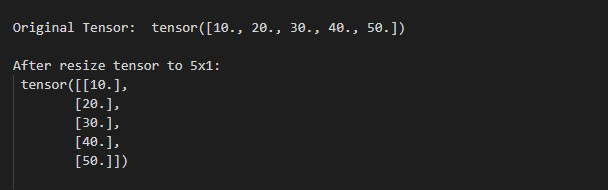My Personal Notes arrow_drop_up
Related Articles